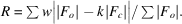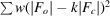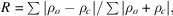International
Tables for
Crystallography
Volume F
Crystallography of biological macromolecules
Edited by M. G. Rossmann and E. Arnold

International Tables for Crystallography (2006). Vol. F. ch. 21.1, pp. 504-505   | 1 | 2 |

## Section 21.1.7.4. Model versus experimental data

G. J. Kleywegta*

aDepartment of Cell and Molecular Biology, Uppsala University, Biomedical Centre, Box 596, SE-751 24 Uppsala, Sweden
Correspondence e-mail: gerard@xray.bmc.uu.se

| top | pdf |

#### 21.1.7.4.1. R values

| top | pdf |

The traditional statistic used to assess how well a model fits the experimental data is the crystallographic R value,This statistic is closely related to the standard least-squares crystallographic residualand its value can be reduced essentially arbitrarily by increasing the number of parameters used to describe the model (e.g. by refining anisotropic ADPs and occupancies for all atoms) or, conversely, by reducing the number of experimental observations (e.g. through resolution and σ cutoffs) or the number of restraints imposed on the model. Therefore, the conventional R value is only meaningful if the number of experimental observations and restraints greatly exceeds the number of model parameters. In 1992, Brünger introduced the free R value (R free; Brünger, 1992a, 1993, 1997; Kleywegt & Brünger, 1996), whose definition is identical to that of the conventional R value, except that the free R value is calculated for a small subset of reflections that are not used in the refinement of the model. The free R value, therefore, measures how well the model predicts experimental observations that are not used to fit the model (cross-validation). Until a few years ago, a conventional R value below 0.25 was generally considered to be a sign that a model was essentially correct (Brändén & Jones, 1990). While this is probably true at high resolution, it was subsequently shown for several intentionally mistraced models that these can be refined to deceptively low conventional R values (Jones et al., 1991; Kleywegt & Jones, 1995b; Kleywegt & Brünger, 1996). Brünger suggests a threshold value of 0.40 for the free R value, i.e. models with free R values greater than 0.40 should be treated with caution (Brünger, 1997). Tickle and coworkers have developed methods to estimate the expected value of R free in least-squares refinement (Tickle et al., 1998). Since the difference between the conventional and free R value is partly a measure of the extent to which the model over-fits the data (i.e. some aspects of the model improve the conventional but not the free R value and are therefore likely to fit noise rather than signal in the data), this difference R freeR should be small (Kleywegt & Jones, 1995a; Kleywegt & Brünger, 1996). Alternatively, the R free ratio (defined as R free/R; Tickle et al., 1998) should be close to unity. Various practical aspects of the use of the free R value have been discussed by Kleywegt & Brünger (1996) and by Brünger (1997).

Self-validation is an alternative to cross-validation and in the case of crystallographic refinement, the Hamilton test (Hamilton, 1965) is a prime example of this. This method enables one to assess whether a reduction in the R value is statistically significant given the increase in the number of degrees of freedom. Application of this test in the case of macromolecules is compounded by the difficulty of estimating the effect of the combined set of restraints on the (effective) number of degrees of freedom, but some information can nevertheless be gained from such an analysis (Bacchi et al., 1996).

#### 21.1.7.4.2. Real-space fits

| top | pdf |

The fit of a model to the data can also be assessed in real space, which has the advantage that it can be performed for arbitrary sets of atoms (e.g. for every residue separately). Jones et al. (1991) introduced the real-space R value, which measures the similarity of a map calculated directly from the model (ρ c) and one which incorporates experimental data (ρ o) aswhere the sums extend over all grid points in the map that surround the selected set of atoms. The real-space fit can also be expressed as a correlation coefficient (Jones & Kjeldgaard, 1997), which has the advantage that no scaling of the two densities is necessary. Chapman (1995) described a modification in which the density calculated from the model is derived by Fourier transformation of resolution-truncated atomic scattering factors.

The program SFCHECK (Vaguine et al., 1999) implements several variations on the real-space fit. The normalized average displacement measures the tendency of groups of atoms to move away from their current position. The density correlation is a modification of the real-space correlation coefficient. The residue-density index is calculated as the geometric mean of the density values of a set of atoms, divided by the average density of all atoms in the model. It therefore measures how high the electron-density level is for the set of atoms considered (e.g. all side-chain atoms of a residue). The connectivity index is identical to the residue-density index, but is calculated only for the N, Cα and C atoms. It thus provides an indication of the continuity of the main-chain electron density.

#### 21.1.7.4.3. Coordinate error estimates

| top | pdf |

Since a measurement without an error estimate is not a measurement, crystallographers are keen to assess the estimated errors in the atomic coordinates and, by extension, in the atomic positions, bond lengths etc. In principle, upon convergence of a least-squares refinement, the variances and covariances of the model parameters (coordinates, ADPs and occupancies) may be obtained through inversion of the least-squares full matrix (Sheldrick, 1996; Ten Eyck, 1996; Cruickshank, 1999). In practice, however, this is seldom performed as the matrix inversion requires enormous computational resources. Therefore, one of a battery of (sometimes quasi-empirical) approximations is usually employed.

For a long time, the elegant method of Luzzati (1952) has been used for a different purpose (namely, to estimate average coordinate errors of macromolecular models) than that for which it was developed (namely, to estimate the positional changes required to reach a zero R value, using several assumptions that are not valid for macromolecules; Cruickshank, 1999). A Luzzati plot is a plot of R value versus, and a comparison with theoretical curves is used to estimate the average positional error. Considering the problems with conventional R values (discussed in Section 21.1.7.4.1), Kleywegt et al. (1994) instead plotted free R values to obtain a cross-validated error estimate. This intuitive modification turned out to yield fairly reasonable values in practice (Kleywegt & Brünger, 1996; Brünger, 1997). Read (1986, 1990) estimated coordinate error from σ A plots; the cross-validated modification of this method also yields reasonable error estimates (Brünger, 1997).

Cruickshank, almost 50 years after his work on the precision of small-molecule crystal structures (Cruickshank, 1949), introduced the diffraction-component precision index (DPI; Dodson et al., 1996; Cruickshank, 1999) to estimate the coordinate or positional error of an atom with a B factor equal to the average B factor of the whole structure. In several cases for which full-matrix error estimates are available, the DPI gives quantitatively similar results. SFCHECK (Vaguine et al., 1999) calculates both the DPI and Cruickshank's 1949 statistic (now termed the `expected maximal error') based on the slope and the curvature of the electron-density map.

#### 21.1.7.4.4. Noncrystallographic symmetry

| top | pdf |

Despite the multitude of criteria for assessing conformational differences between related molecules, there was until recently no objective way to assess whether such differences were a true reflection of the experimental data or a manifestation of refinement artifacts (Kleywegt & Jones, 1995b; Kleywegt, 1996). However, it has been found that electron-density maps calculated with experimental phases (or, at least, phases that are biased as little as possible by the model) and amplitudes can be used to correlate expected similarities (based on the data) with observed ones (manifest in the final refined models; Kleywegt, 1999). This method uses a local density-correlation map, as introduced by Read (Vellieux et al., 1995), to measure the local similarity of the density of two or more models on a per-atom or per-residue basis. By comparing these values to the observed structural differences in the final models, it is relatively easy to check if the latter differences are warranted by the information contained in the experimental data (Kleywegt, 1999).

#### 21.1.7.4.5. Difference density quality

| top | pdf |

van den Akker & Hol (1999) described a method (called DDQ, standing for difference density quality) to assess the local and global quality of a model based on analysis of an (FoFc, α c) map calculated after omission of all water molecules. In this method, the map and model are used to calculate several scores. One score assesses the presence or absence of favourably positioned water peaks near polar and apolar atoms. Other scores provide a measure for the presence or absence of positive and negative shift peaks that may indicate incorrect coordinates, temperature factors or occupancies. The scores can be averaged per residue or for an entire model and can be used to detect problems in models. The method appears to be applicable to ∼3 Å resolution.

### ReferencesAkker, F. van den & Hol, W. G. J. (1999). Difference density quality (DDQ): a method to assess the global and local correctness of macromolecular crystal structures. Acta Cryst. D55, 206–218.Google ScholarBacchi, A., Lamzin, V. S. & Wilson, K. S. (1996). A self-validation technique for protein structure refinement: the extended Hamilton test. Acta Cryst. D52, 641–646.Google ScholarBrändén, C.-I. & Jones, T. A. (1990). Between objectivity and subjectivity. Nature (London), 343, 687–689.Google ScholarBrünger, A. T. (1992a). Free R value: a novel statistical quantity for assessing the accuracy of crystal structures. Nature (London), 355, 472–475.Google ScholarBrünger, A. T. (1993). Assessment of phase accuracy by cross validation: the free R value. Methods and applications. Acta Cryst. D49, 24–36.Google ScholarBrünger, A. T. (1997). The free R value: a more objective statistic for crystallography. Methods Enzymol. 277, 366–396.Google ScholarChapman, M. S. (1995). Restrained real-space macromolecular atomic refinement using a new resolution-dependent electron-density function. Acta Cryst. A51, 69–80.Google ScholarCruickshank, D. W. J. (1949). The accuracy of electron-density maps in X-ray analysis with special reference to dibenzyl. Acta Cryst. 2, 65–82.Google ScholarCruickshank, D. W. J. (1999). Remarks about protein structure precision. Acta Cryst. D55, 583–601.Google ScholarDodson, E., Kleywegt, G. J. & Wilson, K. S. (1996). Report of a workshop on the use of statistical validators in protein X-ray crystallography. Acta Cryst. D52, 228–234.Google ScholarHamilton, W. C. (1965). Significance tests on the crystallographic R factor. Acta Cryst. 18, 502–510.Google ScholarJones, T. A. & Kjeldgaard, M. (1997). Electron density map interpretation. Methods Enzymol. 277, 173–208.Google ScholarJones, T. A., Zou, J.-Y., Cowan, S. W. & Kjeldgaard, M. (1991). Improved methods for building protein models in electron density maps and the location of errors in these models. Acta Cryst. A47, 110–119.Google ScholarKleywegt, G. J. (1996). Use of non-crystallographic symmetry in protein structure refinement. Acta Cryst. D52, 842–857.Google ScholarKleywegt, G. J. (1999). Experimental assessment of differences between related protein crystal structures. Acta Cryst. D55, 1878–1884.Google ScholarKleywegt, G. J., Bergfors, T., Senn, H., Le Motte, P., Gsell, B., Shudo, K. & Jones, T. A. (1994). Crystal structures of cellular retinoic acid binding proteins I and II in complex with all-trans-retinoic acid and a synthetic retinoid. Structure, 2, 1241–1258.Google ScholarKleywegt, G. J. & Brünger, A. T. (1996). Checking your imagination: applications of the free R value. Structure, 4, 897–904.Google ScholarKleywegt, G. J. & Jones, T. A. (1995a). Braille for pugilists. In Proceedings of the CCP4 study weekend. Making the most of your model, edited by W. N. Hunter, J. M. Thornton & S. Bailey, pp. 11–24. Warrington: Daresbury Laboratory.Google ScholarKleywegt, G. J. & Jones, T. A. (1995b). Where freedom is given, liberties are taken. Structure, 3, 535–540.Google ScholarLuzzati, V. (1952). Traitement statistique des erreurs dans la determination des structures crystallines. Acta Cryst. 5, 802–810.Google ScholarRead, R. J. (1986). Improved Fourier coefficients for maps using phases from partial structures with errors. Acta Cryst. A42, 140–149.Google ScholarRead, R. J. (1990). Structure-factor probabilities for related structures. Acta Cryst. A46, 900–912.Google ScholarSheldrick, G. M. (1996). Least-squares refinement of macromolecules: estimated standard deviations, NCS restraints and factors affecting convergence. In Proceedings of the CCP4 study weekend. Macromolecular refinement, edited by E. Dodson, M. Moore, A. Ralph & S. Bailey, pp. 47–58. Warrington: Daresbury Laboratory.Google ScholarTen Eyck, L. F. (1996). Full matrix least squares. In Proceedings of the CCP4 study weekend. Macromolecular refinement, edited by E. Dodson, M. Moore, A. Ralph & S. Bailey, pp. 37–45. Warrington: Daresbury Laboratory.Google ScholarTickle, I. J., Laskowski, R. A. & Moss, D. S. (1998). Rfree and the Rfree ratio. I. Derivation of expected values of cross-validation residuals used in macromolecular least-squares refinement. Acta Cryst. D54, 547–557.Google ScholarVaguine, A. A., Richelle, J. & Wodak, S. J. (1999). SFCHECK: a unified set of procedures for evaluating the quality of macromolecular structure-factor data and their agreement with the atomic model. Acta Cryst. D55, 191–205.Google ScholarVellieux, F. M. D. A. P., Hunt, J. F., Roy, S. & Read, R. J. (1995). DEMON/ANGEL: a suite of programs to carry out density modification. J. Appl. Cryst. 28, 347–351.Google Scholar